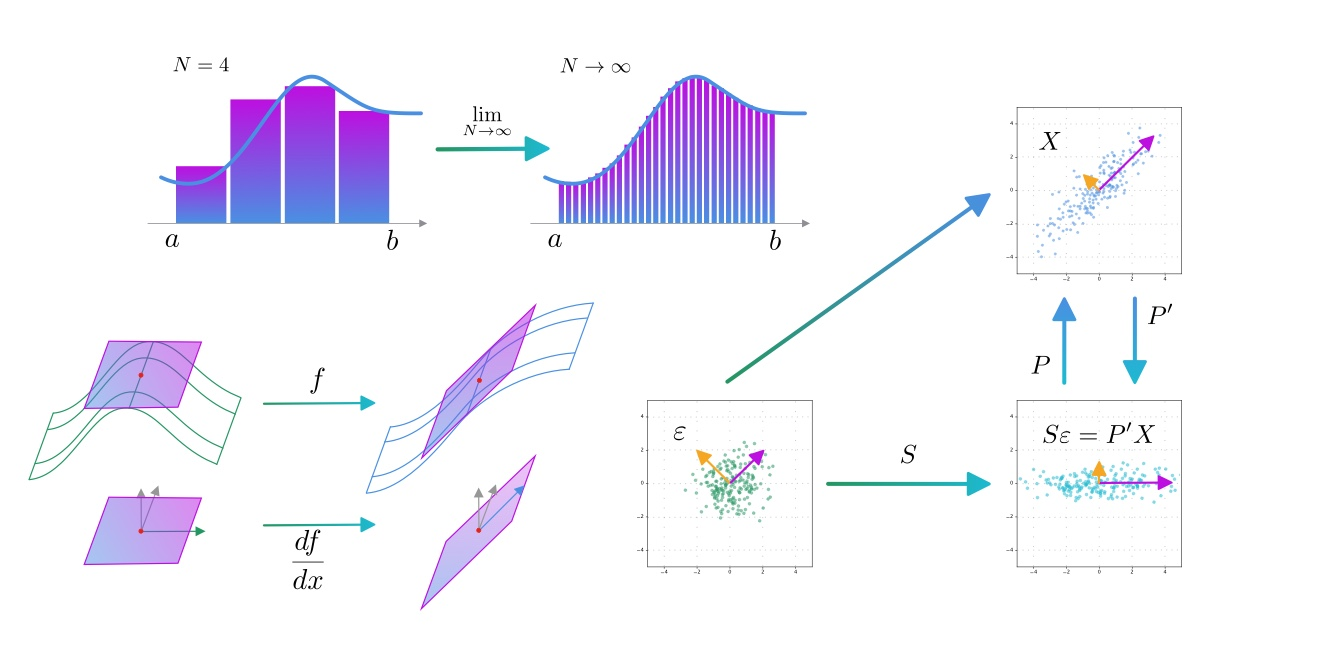# Mathematics for Finance

## University of St.Gallen

2020The course covers some of the basic mathematics required to consume and produce academic research in financial economics. The first topic is linear algebra, including vector spaces, matrix algebra, the dot product and eigen decomposition. Geometrical interpretation of these concepts are provided, together with example applications. Then, it reviews real‑valued random variables and random vectors, exploiting some results and facts from the previous topic. Next, it recalls the definition and meaning of derivative and extend it to multi‑variate vector‑valued functions, again leveraging on linear algebra. Building on that, it then discusses constrained optimization from a theoretical and a practical perspective with a focus on the economic interpretation of Lagrange multipliers. It then moves to more advanced topics like stochastic calculus and stochastic differential equations. In particular, it reviews Itoʹs lemma, risk‑neutral probability. Moreover, an overview of the most relevant SDEs for applications in finance is provided and solution methods ar presented. Finally, it addresses dynamic optimization problems and the Bellman equations, with an eye toward applications in modeling the behavior of market participants.

<<< Back